﻿ Mathematical Formula E=mc2 Squared Written On A Blue, Relatively Dirty Chalkboard By Chalk Stock Image - Image of chalkboard, design: 142357103

# Mathematical formula e=mc2 squared written on a blue, relatively dirty chalkboard by chalk.

## Mathematical formula e=mc2 squared written on a blue, relatively dirty chalkboard by chalk

Photo Taken On: February 04th, 2013

More similar stock images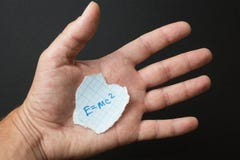The formula E = mc2 in the hand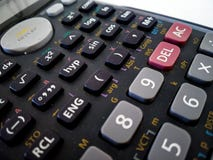close up of scientific calculator isolated with white backgroundPlus icon magical glassy sunburst blue button sky blue background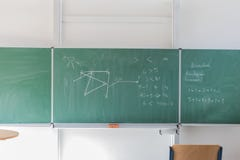Chalkboard with mathematical formula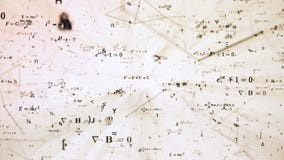Digital futuristic illustration with math, physics formulas in mesh network gridCat in glasses near a fence 2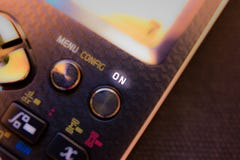Power button ON Key on the keyboard of a scientific calculator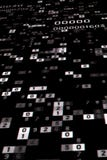mathematical calculation number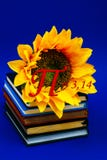Number pi sun flower growing book diary notebook golden section mathematics physics coil figure count school day march blue
Extended licenses